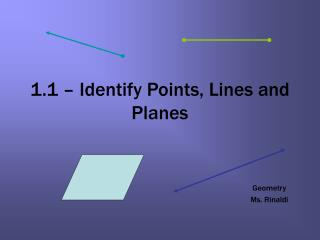DownloadDownload Presentation1.1 – Identify Points, Lines and Planes

# 1.1 – Identify Points, Lines and Planes

Télécharger la présentation## 1.1 – Identify Points, Lines and Planes

- - - - - - - - - - - - - - - - - - - - - - - - - - - E N D - - - - - - - - - - - - - - - - - - - - - - - - - - -
##### Presentation Transcript

1. 1.1 – Identify Points, Lines and Planes Geometry Ms. Rinaldi

2. Undefined Terms The words below have no formal definition, and therefore are called undefined terms. There is agreement as to their meaning though.

3. Definitions • Collinear Points – • Coplanar Points –

4. a. Give two other names for PQand for plane R. b.Name three points that are collinear. Name four points that are coplanar. EXAMPLE 1 Name points, lines, and planes

5. 1. Use the diagram in Example 1. Give two other names for ST. Name a point that is not coplanar with points Q, S, and T. Name points, lines and planes EXAMPLE 2

6. Definitions Segments and rays are collinear if they lie on the same line. So, opposite rays are collinear. Lines, segments, and rays are coplanar if they lie in the same plane.

7. a. Give another name for GH . EXAMPLE 3 Name segments, rays, and opposite rays b. Name all rays with endpoint J . Which of these rays are opposite rays?

8. a. Give another name for EF b. Are HJand JH the same ray ? Are HJand HGthe same ray? Explain. Name segments, rays, and opposite rays EXAMPLE 4

9. Definition • Intersection –

10. c. a. b. EXAMPLE 5 Sketch intersections of lines and planes a. Sketch a plane and a line that is in the plane. b.Sketch a plane and a line that does not intersect the plane. c. Sketch a plane and a line that intersects the plane at a point. SOLUTION

11. STEP 1 Draw: a vertical plane. Shade the plane. STEP 2 Draw: a second plane that is horizontal. Shade this plane a different color. Use dashed lines to show where one plane is hidden. STEP 3 Draw: the line of intersection. EXAMPLE 6 Sketch intersections of planes Sketch two planes that intersect in a line. SOLUTION

12. Sketch intersections of planes EXAMPLE 7 Sketch two different lines that intersect a plane at the same point.

13. Use the diagram at the right. a. Name the intersection of PQand line k. Sketch intersections of planes EXAMPLE 8 b. Name the intersection of plane A and plane B. c. Name the intersection of line kand plane A.

14. Homework Pages 5 – 6 # 3-11, 13, 15-20, 22, 23, 25